# Liquid Solutions - strength of solution - Methods of Expressing the Strength of Solution

Introduction

A solution is a homogeneous mixture of two (or more) substances, the composition of which may vary between certain limits. This concept is valid if we take the example of alcohol and water i.e. both the substances are in same phase.

But what about sugar syrup. Which contains 60% sugar and 40% water? Will the sugar be the solvent? Answers is No, Still the sugar is solute. Then what is the right definition for this case. Well, for hetrogenous substance,

“Solvent is that component of the binary mixture which is in some physical state as the solution will be”.

A solution consisting of two components is called binary solution. The component which is present in large quantity is called solvent and the component which is small in quantity is called solute. If both components are in same physical state.

Methods of Expressing the Strength of Solution

Mass Percentage: It may be defined as number of parts by mass of solute per hundred parts by mass of solution.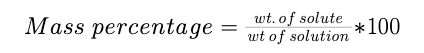Volume Percentage: It may be defined as number of parts by volume of solute per hundred parts by volume of solution.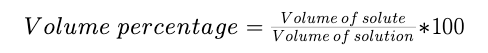Molality: Molality of a solution is defined as the number of moles of solute dissolved in 1 Kg of the solvent.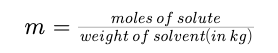Molarity: The molarity of a solution gives the number of gram molecules of the solute present in one litre of the solution. Thus, if one gram molecule of a solute is present in 1 litre of the solution, the concentration of the solution is said to be one molar.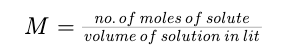Normality: The normality of a solution gives the number of gram equivalents of the solute present in one litre of the solution. Thus, if one gram equivalent of a solute is present in one litre of the solution, the concentration of the solution is said to be one normal.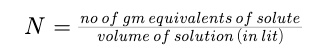Mole Fraction: The mole fraction of any component in a solution is the ratio of the number of moles of that component to the total number of moles of all components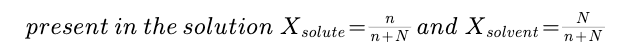n = moles of solute and N = moles of solvent.

Note: Sum of the mole fractions of all the components in the solution is equal to unity.

Example :

Concentrated hydrochloric acid contains 37% (by mass) HCl. The density of its solution is 1.18 g/ml. Calculate the molarity and molality of the solution.

Solution :

Mass of the solution = 100 g
Since density,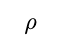= mass / volume
Volume of the solution = mass / density = 100 g/ 1.18 g ml–1 =85ml=85cm3 =0.085dm3

n(HCl) = 37 g/36.5 g mol–1 = 1.01 mol
Molarity of HCl = 1.01 mol/0.085 dm3 = 12.0 mol dm–3 = 12.0 M Mass of solvent = (100 – 37) = 63 g = 0.063 kg
Molality of HCl = 1.01 mol/0.063 kg = 16.03 mol kg–1 = 16.03 m

Example :

Calculate the molality, molarity and mole fraction of ethanol in a solution of total volume 95 ml prepared by adding 50 ml ethanol (density = 0.789 g/ml) to 50 ml water (density = 1.00 g/ml). Also calculate the molality of water in alcohol.

Solution :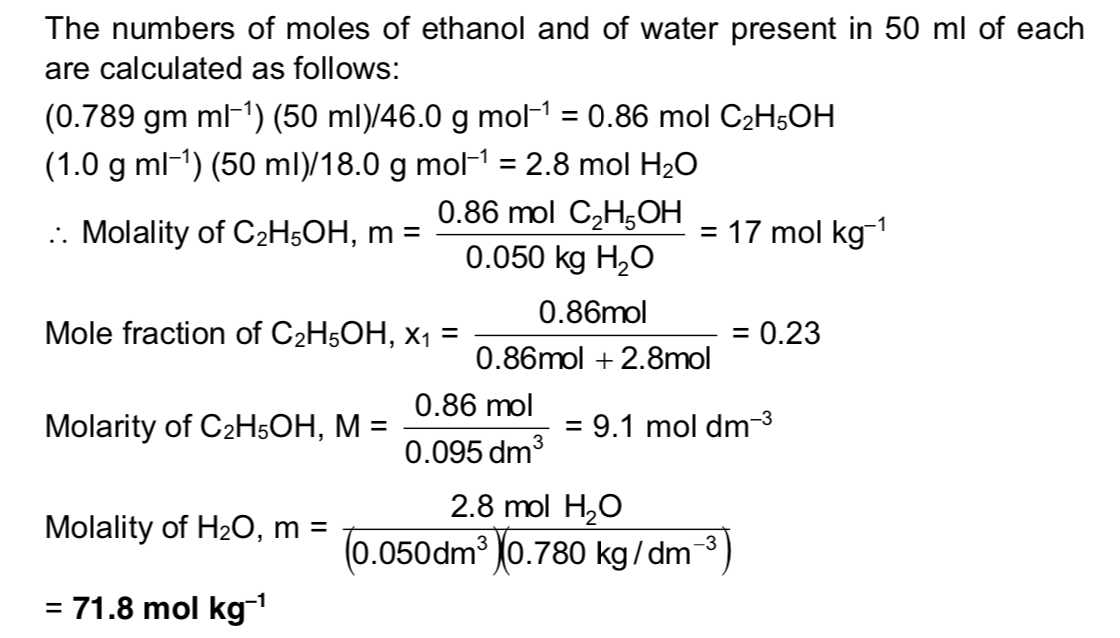Vapour Pressure of Solution

Consider a pure liquid in a beaker is covered with a jar. Some molecules on the liquid surface evaporate and fill the vacant space available to them. The molecules in the vapour phase move randomly in the vacant space, during this motion, they strike the surface of the liquid and condensed. This process of evaporation and condensation go on and an equilibrium is established between evaporation and condensation. The pressure exerted by vapours over the liquid surface at equilibrium is called vapour pressure of the liquid.

If solute is non volatile solid or liquid the vapour pressure of solution is equal to partial vapour pressure of solvent in the solution and if the solute is volatile solid or liquid, then vapour pressure will be equal to the sum of partial vapour pressure of solute and that of solvent.

Raoult’s Law

This law is applied for a solution of liquid in liquids and can be stated as follows.

“ The partial vapour pressure of any component in the solution is directly proportional to its mole fraction”.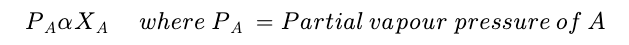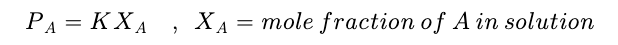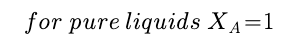Then K = PAo Where PAo is the vapour pressure of component A in pure state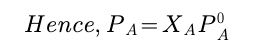Similarly for component B,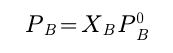Raoult’s Law in Combination with Dalton’s Law of Partial Pressure: Assuming that vapours of a liquid is behaving like an ideal gas, then according to Dalton’s law of partial pressure the total pressure PT is given by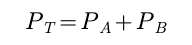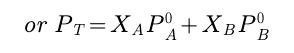Composition of the Vapour: The composition of the liquid and vapour that are in mutual equilibrium are not necessarily the same, the common sense suggest that the vapour pressure should be richer in the more volatile component. This expectation can be confirmed as follows:

Let the mole fractions of A and B in vapour phase be YA and YB then from Dalton’s law,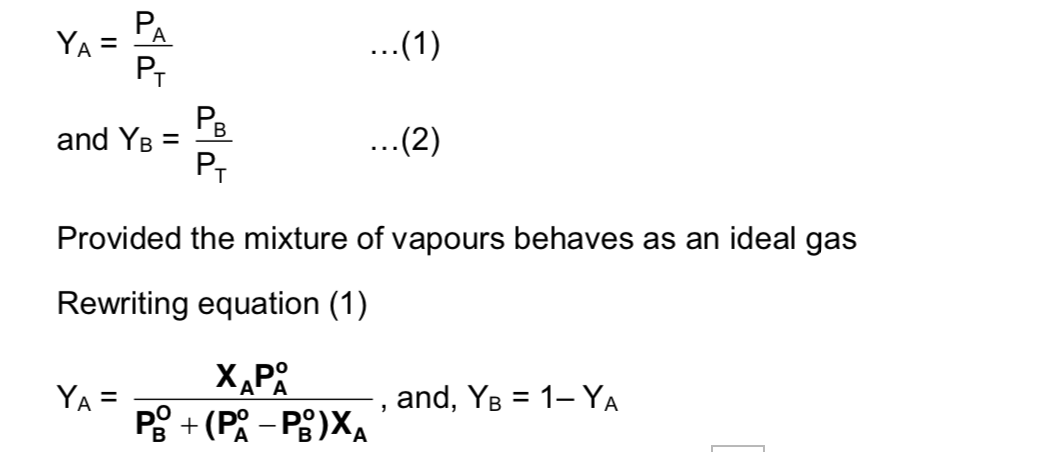Example :

The vapour pressures of pure benzene and toluene at 40°C are 184.0 torr and 59.0 torr, respectively. Calculate the partial pressures of benzene and toluene, the total vapour pressure of the solution and the mole fraction of benzene in the vapour above the solution that has 0.40 mole fraction of benzene. Assume that the solution is ideal.

Solution :

Let us designate benzene by subscript A and toluene by subscript B. In solution xA = 0.40 and hence XB = 0.6

PA = xAA = (0.40) (184.0 torr) = 73.6 torr

PB = xBB = (0.60) (59.0 torr) = 35.4 torr

P=PA +PB =73.6torr+35.4torr=109.0torr

XA, vap = PA/P = PA/(PA + PB) = 73.6 torr/109.0 torr = 0.675

Condensation of vapours of solution: When the vapours of solution (containing liquids A and B) is condensed, the composition of liquids A and B in the condensate remains same. The vapours over condensate can again recondensed and the composition of A and B in condenstate (2) remains same as it was in vapour phase over condensate (2).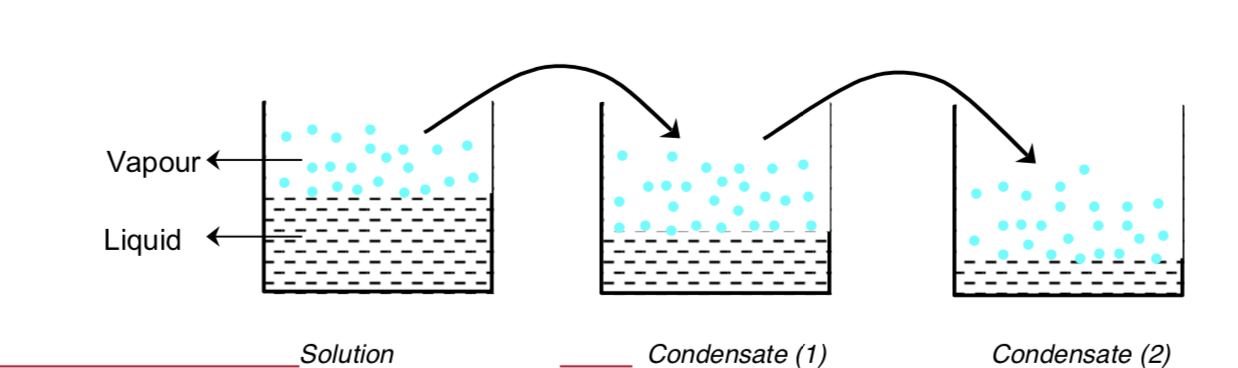Example :

Liquids A and B form an ideal solution. The vapour pressure of A and B at 100°C are 300 and 100 mm Hg respectively. Suppose that vapour above solution is composed of 1 mole of A and 1 mole of B is collected and condensed. This condensate is then heated at 100°C and vapour are again condensed to form a liquid L. What is the mole fraction of A in the vapours of L?

Solution:

Vapour pressure due to vapours above solution PT=XAPAo +XBPBo;

PT = 300XA + 100 XB

It is given that in vapour phase each of A and B are one mole each hence each of them have mole fraction 0.5 in vapour phase

0.5 = 300XA ...(1) PT

0.5 = 100XB ...(2) PT

andXA+XB =1 ...(3)

Solving equation (1), (2) and (3), we get

XA = 0.25 and XB = 0.75

PT= 300 0.25 + 100 0.75 = 75 + 75 = 150 mm

After condensation of vapours

In condensate (1)

XA =0.5XB =0.5

PT =0.5300+0.5100=150 +50=200mm Mole fraction of A & B in vapour phase of condensate

YA=PA =150=0.75; P 200

YB =1–0.75=0.25

When the vapours of the condensate (1) will again be vapourised in condensate (2) liquid L

XA = 0.75 XB = 0.25, where XA and XB are mole fractions of A and B in liquid L

PT=3000.75 +1000.25=225+25=250mm

and mole fraction of A in vapour phase of the condensate (2) is given by

YA = 225 = 0.9 250

Post By : Rahul Kumar 13 Mar, 2020 2883 views Chemistry